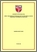# Direct one-step block methods for solving special second order delay differential equations

## Citation

Rasdi, Nadzirah (2013) Direct one-step block methods for solving special second order delay differential equations. Masters thesis, Universiti Putra Malaysia.

## Abstract

This thesis describes the implementation of one-step block methods of Runge-Kutta type for solving second order Delay Differential Equations (DDEs). The one-step block method will solve the second order DDEs directly without reducing to first order equations. The solution will be approximated simultaneously at more than one point based on equidistant block method and each of these values is calculated independently of each other. In this research, the block methods are implemented based on the predictor-corrector scheme using constant step size technique. The derivation of two point, three point, extended two point and extended three point implicit block methods were also described. Those methods were derived using Lagrange interpolation polynomial. The stability polynomials for the methods are obtained and their regions of absolute stability are discussed. Subsequently, the one-step block methods were then adapted to solve delay differential equations. The basic idea is to apply the same integration method and use interpolation polynomial to approximate the solution in the previous value. The efficiency of the proposed block method is supported by some numerical results. The results showed that the block methods proposed in this thesis are suitable to solve second order DDEs.Preview
PDF
FS 2013 38 IR.pdfView Item